# Graphing Logarithmic Functions

The function $y={\mathrm{log}}_{b}x$ is the inverse function of the exponential function $y={b}^{x}$ .

Consider the function $y={3}^{x}$ . It can be graphed as: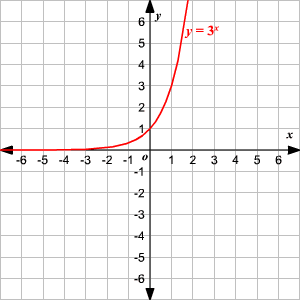The graph of inverse function of any function is the reflection of the graph of the function about the line $y=x$ . So, the graph of the logarithmic function $y={\mathrm{log}}_{3}\left(x\right)$ which is the inverse of the function $y={3}^{x}$ is the reflection of the above graph about the line $y=x$ .

$\begin{array}{|cccccccc|}\hline x& \frac{1}{9}& \frac{1}{3}& 1& 3& 9& 27& 81\\ y={\mathrm{log}}_{3}x& -2& -1& 0& 1& 2& 3& 4\\ \hline\end{array}$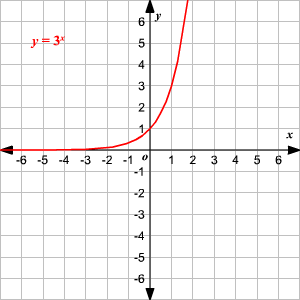The domain of the function is the set of all positive real numbers.

When no base is written, assume that the log is base $10$ .

$\begin{array}{|cccccccc|}\hline x& \frac{1}{1000}& \frac{1}{100}& \frac{1}{10}& 1& 10& 100& 1000\\ y=\mathrm{log}x& -3& -2& -1& 0& 1& 2& 3\\ \hline\end{array}$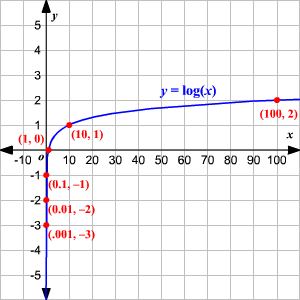The logarithmic function, $y={\mathrm{log}}_{b}\left(x\right)$ , can be shifted $k$ units vertically and $h$ units horizontally with the equation $y={\mathrm{log}}_{b}\left(x+h\right)+k$ .

Vertical shift

If  $k>0$ , the graph would be shifted upwards.

If  $k<0$ , the graph would be shifted  downwards.

Horizontal Shift

If  $h>0$ , the graph would be shifted  left.

If  $h<0$ , the graph would be shifted  right.

Consider the logarithmic function $y=\left[{\mathrm{log}}_{2}\left(x+1\right)-3\right]$ . This can be obtained by translating the parent graph $y={\mathrm{log}}_{2}\left(x\right)$ a couple of times.

Consider the graph of the function $y={\mathrm{log}}_{2}\left(x\right)$ .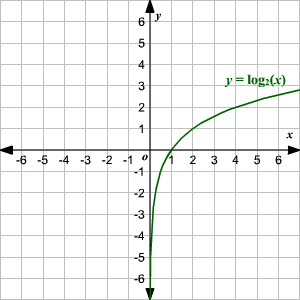Since $h=1$ , $y=\left[{\mathrm{log}}_{2}\left(x+1\right)\right]$ is the translation of $y={\mathrm{log}}_{2}\left(x\right)$ by one unit to the left.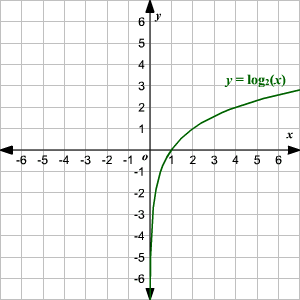Now, $k=-3$ . The graph of $y=\left[{\mathrm{log}}_{2}\left(x+1\right)\right]$ will be shifted $3$ units down to get $y=\left[{\mathrm{log}}_{2}\left(x+1\right)\right]-3$ .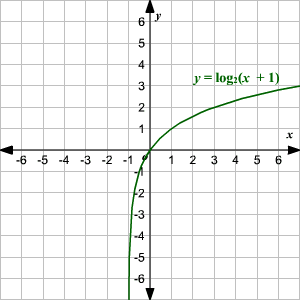You may recall that logarithmic functions are defined only for positive real numbers. This is because, for negative values, the associated exponential equation has no solution. For example, ${3}^{x}=-1$ has no real solution, so ${\mathrm{log}}_{3}\left(-1\right)$ is undefined.

So, what about a function like $y={\mathrm{log}}_{4}\left(-x\right)$ ?

This is defined only for negative values of $x$ .

Find the values of the function for a few negative values of $x$ . For an easier calculation you can use the exponential form of the equation, ${4}^{y}=-x$ .

$\begin{array}{|ccccccc|}\hline x& -1& -2& -4& -8& -16& -32\\ \begin{array}{l}y={\mathrm{log}}_{4}\left(-x\right)\\ \text{or}\\ {4}^{y}=-x\end{array}& 0& \frac{1}{2}& 1& 1\frac{1}{2}& 2& 2\frac{1}{2}\\ \hline\end{array}$

Plot the points and join them by a smooth curve.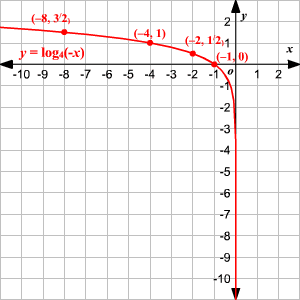You can see that the graph is the reflection of the graph of the function $y={\mathrm{log}}_{4}\left(x\right)$ about the $y$ -axis.